## Example Questions

### Example Question #1 : How To Find A Rational Number From An Exponent

Quantitative Comparison: Compare Quantity A and Quantity B, using additional information centered above the two quantities if such information is given.

Quantity A             Quantity B

43                              34

The two quantities are equal.

Quantity A is greater.

Quantity B is greater.

The answer cannot be determined from the information given.

Quantity B is greater.

Explanation:

In order to determine the relationship between the quantities, solve each quantity.

4is 4 * 4 * 4 = 64

34 is 3 * 3 * 3 * 3 = 81

Therefore, Quantity B is greater.

### Example Question #11 : Exponents And Rational Numbers

Quantity A: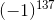Quantity B:The relationship cannot be determined from the information given.

Quantity B is greater.

Quantity A is greater.

The two quantities are equal.

Quantity B is greater.

Explanation:

(–1) 137= –1

–1 < 0

(–1) odd # always equals –1.

(–1) even # always equals +1.

### Example Question #31 : Algebra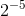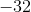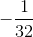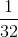Explanation:

Anything raised to negative power meansover the base raised to the postive exponent.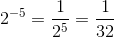### Example Question #32 : Algebra

Which of the following is not the same as the others?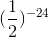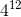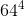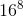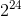Explanation:

Let's all convert the bases to.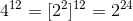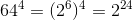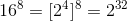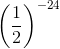This one may be intimidating but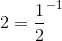.

Therefore,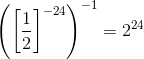is not like the answers so this is the correct answer.

### Example Question #5 : How To Find A Rational Number From An Exponent

Simplify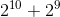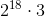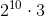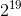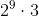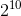Explanation:

Whenever you see lots of multiplication (e.g. exponents, which are notation for repetitive multiplication) separated by addition or subtraction, a common way to transform the expression is to factor out common terms on either side of the + or - sign. That allows you to create more multiplication, which is helpful in reducing fractions or in reducing the addition/subtraction to numbers you can quickly calculate by hand as you'll see here.

So let's factor a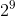.

We have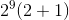.

And you'll see that the addition inside parentheses becomes quite manageable, leading to the final answer ofTired of practice problems?

Try live online GRE prep today.